# Neural Networks - Exposing the Hidden Layer¶

### This tutorial will conver what hidden layers actually are doing with your data when they are trying to learn a decision boundary.¶

In artificual neural networks there is the concept of the hidden layer. Traditionally what is done in these hidden layers is obfuscated from the user, some people even refer to the operations done by the hidden layer as magic. I don't like explanations that do that because it leads to people thinking of neural networks as a black box as opposed to intelligently thinking about how to design these networks to suit their needs.

This lesson is intended for people who already have some idea what neural networks are and what they do but may not know the nuances of how neural networks really function.

Now, with that introduction out of the way, lets look at some data

In :
import matplotlib.pyplot as plt
import random

#Lets generate some data, this data will be in the form of 2D points between -1 and 1
#a circle contained within this range will be classified as label -1 and everything else will be labeled as 1
def generateNested2DData(numSamples):
xy1 = {"x":[],"y":[]}                                # Create some data structures to store our data
xy2 = {"x":[],"y":[]}
for i in range(0, numSamples):
x = (random.random()*2)-1                        # Generate a random datapoint between -1 and 1 for x
y = (random.random()*2)-1                        # and for y
origin_dist = ((x**2) + y**2)**.5                # Calculate the distance of that point from (0,0)
label = 1 if origin_dist > ((2)**.5)/2.0 else -1 # if the distance is > than a threshold assign label 1 otherwise -1

if(label==1):                                    # Assign the data to our data structure
xy1["x"].append(x)
xy1["y"].append(y)
else:
xy2["x"].append(x)
xy2["y"].append(y)

return xy1, xy2

labelA, labelB = generateNested2DData(500)                    # Generate 500 datapoints in the way that we described

plt.scatter(labelA["x"], labelA["y"], marker='x', color="r")
plt.scatter(labelB["x"], labelB["y"], marker='o', color="b")
plt.show()                                                    # Plot them to see what it looks like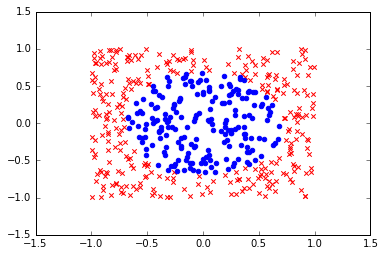Now the data here is clearly not linearly seperable. If you tried to find a line where all of the blue points are on one side of the line and all of the red points are on another you would fail because the blue points are entirely surrounded by the red points.

As a result all the machine learning models for linear seperable data (vanilla SVM, Perceptron, etc) would fail on any of this raw data.

So what do we do if we want to run a simple model on this data? Obviously you want as simple a model as possible to keep your model from overfitting and to keep the bound between error on test and error on training tight, but that doesnt really tell us much other than we should just try and limit our parameters.

But lets think about transformations we can apply to the data...

But lets look at our data and think about it. Can we imagine, if we were to transform our data into 3, 4, 5, or 6 dimensions could we imagine a seperating hyperplane? Well, as you can imagine, its hard to visualize any data beyond the 3rd dimension. But lets think about the 3rd dimension then, I can imagine that if we projected the data onto a cone, with the point of the cone at (0,0) we could find a plane somewhere along the height of the cone that seperates the data linearly.

So the next question is... how do we come up with that transformation? Sure we could write it ourselves, but thats no fun. What if we could learn that transformation?

Lets look at common functions that can be used for data transformation, specifically the weighted average (You will see where I am going with this)

Here we have the function for a weighted average:

## Weighted Average = $\frac{\sum_{i=0}^{N} w_i*f_i} {\sum_{i=0}^{N} w_i}$

This function takes the sum of a series of values, and then multiplies them by some weights and then divides by the sum of the weights to normalize the values into the possible range of values used. Hopefully you are framiliar with this process so I wont go into very much detail... but... this looks an awful lot like a neuron's activation function.

Here we have the function for the in some neuron:

## Neural Activation = $tanh(\sum_{i=0}^{N} w_i*f_i)$

Obviously, you can use a number of different activation functions (tanh, sigmoid, linear, etc) but its the same process as a weighted average. You have a series of values (neuron inputs) which get summed by the products of the respective weights and instead of dividing by the sum of the weights to normalize (as you did with the weighted average) you squash the sum between 1 and -1 (or something else depending on your activation function) in order to normalize.

More over, a layer that has an input of size $N$ with $M$ neurons takes an input of dimensionality $N$ and performs $M$ weighted averages thus transforming the data into $M$ dimensions where each dimension is an individual weighted average.

So lets think about this in terms of what a complete neural network does overall. Each layer can be seen as multiple weighted averages over the inputs, and the entire network can be viewed as successive applications of these multiple weighted averages.

Basically, a hidden layer takes $N$ inputs and projects the data into $M$ output dimensions. If $M$ and $N$ are equal, then you can see this operation taking the data and warping it. This $M$ dimensional transform is commonly refered to as a neural representation.

Now, lets look back at our data and see what happens when we pump the data through different neurons

In :
import matplotlib.pyplot as plt
import numpy as np

labelA, labelB = generateNested2DData(500)                    # Generate 500 datapoints in the way that we described above

plt.scatter(labelA["x"], labelA["y"], marker='x', color="r")
plt.scatter(labelB["x"], labelB["y"], marker='o', color="b")
print("Here is the raw input to our network")
plt.show()                                                    # Plot them to see what it looks like for reference

# So lets see what a simple weighted projection looks like in 2D
# First lets define an activation function, as if we were writing the activation function of a neuron
# This isnt how you should code a full neural network, but it works for our purposes here.
def activate(weights, data):
output = []
for x, y in zip(data["x"], data["y"]):
squashedWeightedSum = np.tanh(weights*x + weights*y)
output.append(squashedWeightedSum)
return output

# Now lets generate a random set of weights, like you would have in a neural network
neuron1Weights = np.random.rand(2)
a1=activate(neuron1Weights, labelA)  # Get the activations for first neuron we created
b1=activate(neuron1Weights, labelB)

plt.scatter(a1, *len(a1), marker='x', color="r")
plt.scatter(b1, *len(b1),  marker='o', color="b")
print("Here is what a randomly initialized 1D hidden layer does to the 2D input to our network")
plt.show()

neuron2Weights = np.random.rand(2)
a2=activate(neuron2Weights, labelA)  # Get the activations for second neuron we created
b2=activate(neuron2Weights, labelB)

#Lets see what the warping looks like
plt.scatter(a1, a2, marker='x', color="r")
plt.scatter(b1, b2,  marker='o', color="b")
print("Here is what a randomly initialized 2D hidden layer does to the 2D input to our network")
plt.show()                                                    # Plot them to see what it looks like for reference

neuron3Weights = np.random.rand(2)
a3=activate(neuron3Weights, labelA)  # Get the activations for the third neuron we need here
b3=activate(neuron3Weights, labelB)

fig = plt.figure()
ax.scatter(a1, a2, a3, marker='x', color="r")
ax.scatter(b1, b2, b3,  marker='o', color="b")
print("Here is what a randomly initialized 3D hidden layer does to the 2D input to our network")
plt.show(fig)                                                # Plot them to see what it looks like for reference

Here is the raw input to our network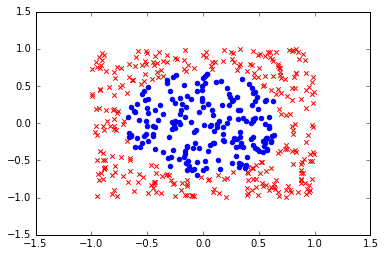Here is what a randomly initialized 1D hidden layer does to the 2D input to our network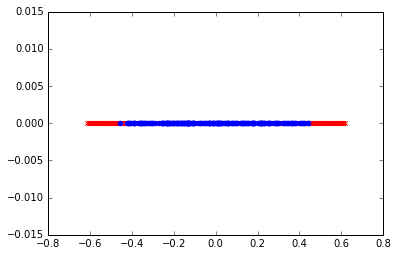Here is what a randomly initialized 2D hidden layer does to the 2D input to our network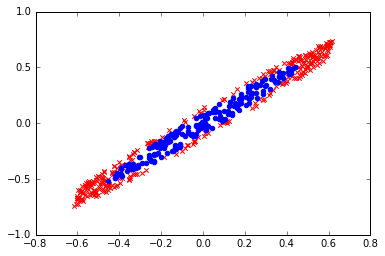Here is what a randomly initialized 3D hidden layer does to the 2D input to our network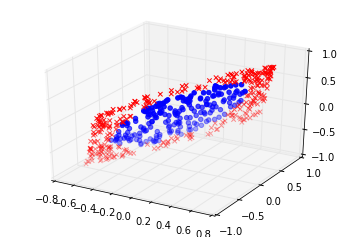Hopefully you now see what hidden layers are actually doing, they are warping/projecting data into a relevant target dimension. In the above case we can see it with 1, 2 and 3 dimensions, but this is how it works in all dimensions. Its just much harder to visualize a high dimensional space.

Now earlier, we imagined that this data could be projected onto a cone for linear classification. What if I told you that learning that cone, is exactly a layer in a neural network with 3 neurons does for this type of data...

Lets imagine training a neural network with shape 2-3-2-1. This means that there is a 2D input (layer 0) which is then projected into 3D for classification (layer 1), which is then projected back down into 2 dimensions (layer 2), which is then finally projected back down into 1 dimension (layer 3), or a single value between -1 and 1 for final classification.

This network is capable of learning this conical shape in order to properly seperate the data. This is due entirely to the 3D transformation that takes place in layer 1. The 2nd layer serves to further seperate the data and demonstrate the effects of the 3D transformation. You can view the training of this network in the image below. The decision boundary for the network is highlighted in green.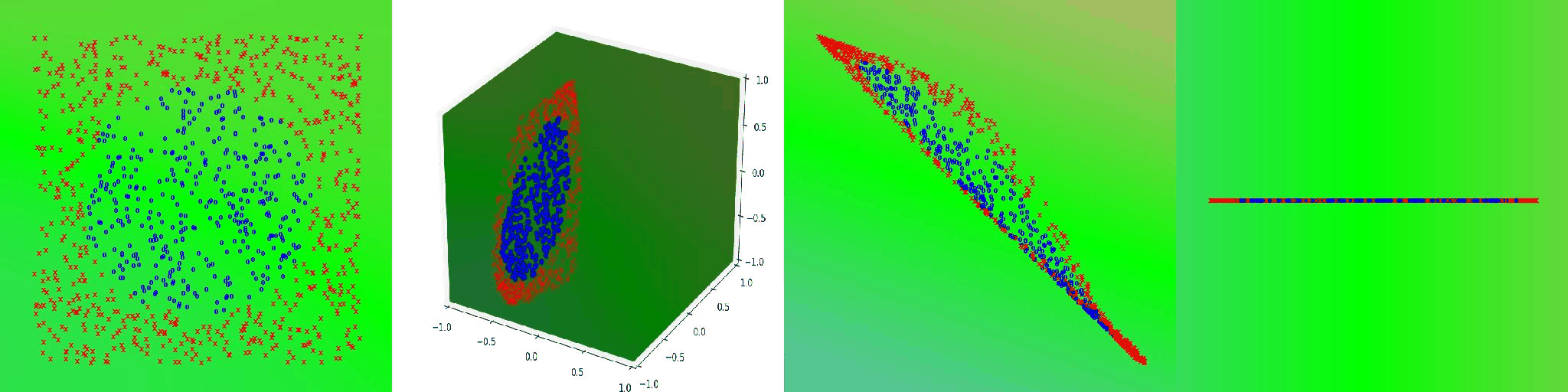###### The first image on the left is the input (layer 0), the second image is the 3D transformation (layer 1), the third image is the 2D transformation (layer 2), and the final image is the 1D transformation (layer 3).

This clearly shows the steps that a neural network goes through in order to learn how to predict a distribution such as this. First the data is projected into the shape of a cone, then the data is projected into 2 dimensions for further optimization, and then finally onto a numberline for final classification.

Hopefully this helps you understand how hidden layers in neural networks really work and why it is very important to choose your neural network architecture ahead of training time. If you don't think hard about your network layout, you will likely overfit or fail to learn the data.

Thanks for taking the time to read this! Hopefully you learned something.

# Appendix¶

Included below are several other visualizations of networks learning seperate distributions.

2-3-2-1 Xor

2-3-2-1 Wavy

2-3-2-1 Yin-Yang

2-3-2-1 Concentric Circles